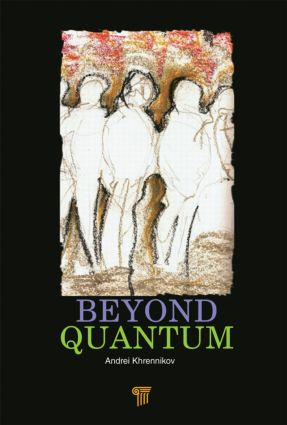# Beyond Quantum

## 1st Edition

Jenny Stanford Publishing

392 pages | 14 B/W Illus.

##### Purchasing Options:\$ = USD
Hardback: 9789814411738
pub: 2014-04-10
\$160.00
x
eBook (VitalSource) : 9780429073663
pub: 2014-04-10
from \$28.98

FREE Standard Shipping!

### Description

The present wave of interest in quantum foundations is caused by the tremendous development of quantum information science and its applications to quantum computing and quantum communication. It has become clear that some of the difficulties encountered in realizations of quantum information processing have roots at the very fundamental level. To solve such problems, quantum theory has to be reconsidered. This book is devoted to the analysis of the probabilistic structure of quantum theory, probing the limits of classical probabilistic representation of quantum phenomena.

Introduction

Author’s views on quantum foundations

Prequantum classical statistical field theory: introduction

Where is discreteness? Devil in detectors?

On experiments to tests the Euclidean model

Conventional quantum theory: fundamentals

Postulates

Quantization

Interpretations of Wave Function

V¨axj¨o interpretation of quantum mechanics

Short introduction to classical probability theory

Quantum Conditional Probability

Interference of Probabilities in Quantum Mechanics

Two slit experiment

Corpuscular interference

Interference of probabilities in cognitive science

Fundamentals of Prequantum Classical Statistical Field Theory

Noncomposite systems

Composite systems

Stochastic process corresponding to Schr¨odinger’s evolution

Correlations of the components of the prequantum field

PCSFT-formalism for classical electromagnetic field-1

Discussion of a possible experimental verification of PCSFT

Photonic field

Correlation between polarization vectors of entangled photons

Functionals of prequantum fields corresponding to operators of photon polarization

Classical representation of Heisenberg’s uncertainty relation

Towards violation of Born’s rule: description of a simple experiment

Why Gaussian?

On correspondence between quantum observables and classical variables

Prequantum Dynamics from Hamiltonian Equations on the Infinite-dimensional Phase Space

Hamiltonian mechanics

Symplectic representation of Schrödinger dynamics

Classical and quantum statistical models

Measures on Hilbert spaces

Lifting of pointwise dynamics to spaces of variables and measures

Dispersion preserving dynamics

Dynamics in the space of physical variables

Probabilistic dynamics

Detailed analysis of dispersion preserving dynamics

Quantum Mechanics as Approximation of Statistical Mechanics of Classical Fields

The Taylor approximation of averages for functions of random variables

Quantum model: finite-dimensional case

Prequantum → quantum correspondence: finite dimensional case

Prequantum phase space: infinite-dimensional case

Gaussian measures corresponding to pure quantum states

Illustration of the prequantum →quantum coupling in the case of qubit mechanics

Prequantum classical statistical field theory (PCSFT)

PCSFT-formalism for classical electromagnetic field-2

Asymptotic expansion of averages with respect to electromagnetic random field

Interpretation

Simulation of quantum-like behavior for the classical electromagnetic field

Maxwell equations as Hamilton equations or as Schrödinger equation

Quadratic variables without quantum counterpart

Generalization of quantum mechanics

Coupling between the time scale and dispersion of a prequantum random signal

Supplementary Mathematical Considerations

Dispersion preserving dynamics with nonquadratic Hamilton functions

Formalism of rigged Hilbert space

Quantum pure and mixed states from the background field

Classical model for unbounded quantum observables

Mathematical Presentation for Composite Systems

Derivation of basic formulas

Vector and operator realizations of the tensor product

Operation of the complex conjugation in the space of self-adjoint operators

The basic operator equality for arbitrary (bounded) self-adjoint operators

Operator representation of reduced density operators

Classical random field representation of quantum correlations

Infinite-dimensional case

Correlations in triparticle systems

PCSFT-representation for a mixed state

Phenomenological Detection Model 271

Finite-dimensional model

Position measurement for the prequantum field

Field’s energy detection model

Coupling between probabilities of detection of classical random fields and quantum particles

Deviation from predictions of quantum mechanics

Averages

Local measurements

Measurement of observables with discrete spectra

Classical field treatment of discarding of noise contribution in quantum detectors

Quantum channels as linear filters of classical signals

Quantum individual events

Classical random signals: ensemble and time representations of averages

Discrete-counts model for detection of classical random signals

Quantum probabilities from threshold type detectors

The case of an arbitrary density operator

The general scheme of threshold detection of classical random signals

Probability of coincidence

Stochastic process description of detection

### About the Editor

Andrei Khrennikov is a professor of applied mathematics at Linnaueus University (Växjö, South-East Sweden) and the director of the multidisciplinary research center at this university, the International Center for Mathematical Modeling in Physics, Engineering, Economics, and Cognitive Science.

### Subject Categories

##### BISAC Subject Codes/Headings:
SCI040000
SCIENCE / Mathematical Physics
SCI041000
SCIENCE / Mechanics / General
SCI057000
SCIENCE / Quantum Theory
SCI086000
SCIENCE / Life Sciences / General